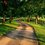# [Differential Geometry] Comparing the Christoffel Symbols for two Parametrisations of the Plane

We can parametrise an open set of the plane using Cartesian coordinates, $$\varphi: U \subset \mathbb{R}^2\rightarrow \mathbb{R}^3$$, where $$\varphi(u,v) = (u,v,0)$$. Then $$\varphi_u = (1,0,0)$$ and $$\varphi_v = (0,1,0).$$ $\implies \varphi_{uu} = (0,0,0) = \varphi_{uv} = \varphi_{vv}.$

So $\Gamma_{ij}^k =0, \forall i,j,k =1,2$.

How about in polar coordinates? We can parametrise an open set of the plane with $\varphi: V \subset \mathbb{R}^2 \rightarrow \mathbb{R}^3$ where $\varphi(u,v) = (u\cos v, u\sin v, 0)$ this time.

Then $\varphi_u = (\cos v, \sin v, 0),$ and $\varphi_v = (-u \sin v, u\cos v, 0),$ and the coefficients of the first fundamental form are $E= \cos^2v+sin^2v=1,$ $F= -u\sin v\cos v+ u\sin v\cos v= 0,$ $G=u^2.$ Considering the trihedron given by the vectors $X_u, X_v, N$, taking inner product with $X_u$, $X_v$, we have the following system of equations:

(for reference, see fifth note on computing Gaussian curvature)

$\Gamma^1_{11}E + \Gamma^2_{11}F = \frac{1}{2} E_u ,$ $\Gamma^1_{11}F + \Gamma^2_{11}G = F_u-\frac{1}{2}E_v.$ $\Gamma^1_{12}E + \Gamma^2_{12}F = \frac{1}{2}E_v,$ $\Gamma^1_{12}F+ \Gamma^2_{12}G = \frac{1}{2} G_u.$ $\Gamma^1_{22}E + \Gamma^2_{22}F = F_v - \frac{1}{2}G_u,$ $\Gamma^1_{22}F + \Gamma^2_{22}G = \frac{1}{2}G_v.$ To find the Christoffel symbols, we let

$\begin{bmatrix} E & F \\ \\ F & G \\ \end{bmatrix} \begin{bmatrix} \Gamma^1_{11} & \Gamma^1_{12} & \Gamma^1_{22}\\ \\ \Gamma^2_{11} & \Gamma^2_{12} &\Gamma^2_{22}\\ \end{bmatrix} = \begin{bmatrix} \frac{1}{2} E_u & \frac{1}{2}E_v & F_v - \frac{1}{2}G_u\\ \\ F_u-\frac{1}{2}E_v & \frac{1}{2} G_u & \frac{1}{2}G_v\\ \end{bmatrix}.$

Hence, $\begin{bmatrix} \Gamma^1_{11} & \Gamma^1_{12} & \Gamma^1_{22}\\ \\ \Gamma^2_{11} & \Gamma^2_{12} &\Gamma^2_{22}\\ \end{bmatrix} = \begin{bmatrix} E & F \\ \\ F & G\\ \end{bmatrix}^{-1} \begin{bmatrix} \frac{1}{2} E_u & \frac{1}{2}E_v & F_v - \frac{1}{2}G_u\\ \\ F_u-\frac{1}{2}E_v & \frac{1}{2} G_u & \frac{1}{2}G_v\\ \end{bmatrix}.$

And since $E_u =0, E_v =0, F_u =0, F_v=0, G_u=2u, G_v=0$, $(from E=1, F=0, G=u^2)$ $RHS = \begin{bmatrix} ~1 & 0 ~ \\ \\ 0 & u^2 ~\\ \end{bmatrix}^{-1} \cdot \begin{bmatrix} ~0 & 0 & -u ~\\ \\ ~0 & u & 0 ~\\ \end{bmatrix}$

$= \begin{bmatrix} ~0 & 0 & -u ~\\ \\ ~0 & \frac{1}{u} & 0 ~\\ \end{bmatrix}.$

Therefore, $\Gamma_{11}^1=0, \Gamma_{12}^1=0, \Gamma_{22}^2=-u, \Gamma_{11}^2=0, \Gamma_{12}^2= \frac{1}{u}, \Gamma_{22}^2=0$.

In fact, we can check that the Gaussian curvature of the open set of the plane is zero $(K \equiv 0)$, by substituting the Christoffel symbols above in the formula for Gauss Curvature:

$-EK = (\Gamma_{12}^2)_u - (\Gamma_{11}^2)_v + \Gamma_{12}^1\Gamma_{11}^2+\Gamma_{12}^2\Gamma_{12}^2-\Gamma_{11}^2\Gamma_{22}^2-\Gamma_{11}^1\Gamma_{12}^2 .$

$-EK = \left(\frac{1}{2}\frac{G_u}{G}\right)_u - \left(-\frac{1}{2}\frac{E_v}{G}\right)_v - \frac{1}{4}\frac{E_u}{E}\frac{G_u}{G} - \frac{1}{4}\frac{E_v}{E}\frac{E_v}{G} +\frac{1}{4}\frac{E_v}{G}\frac{G_v}{G} +\frac{1}{4}\frac{G_u}{G}\frac{G_u}{G}$ $\implies -K = \left(\frac{1}{u}\right)_u -0+0+ \left(\frac{1}{u}\right)\left(\frac{1}{u}\right)-0-0$ $\implies -K = -\frac{1}{u^2} + \frac{1}{u^2} =0,~~\therefore K = 0$Note by Bright Glow
3 years, 1 month ago

This discussion board is a place to discuss our Daily Challenges and the math and science related to those challenges. Explanations are more than just a solution — they should explain the steps and thinking strategies that you used to obtain the solution. Comments should further the discussion of math and science.

When posting on Brilliant:

• Use the emojis to react to an explanation, whether you're congratulating a job well done , or just really confused .
• Ask specific questions about the challenge or the steps in somebody's explanation. Well-posed questions can add a lot to the discussion, but posting "I don't understand!" doesn't help anyone.
• Try to contribute something new to the discussion, whether it is an extension, generalization or other idea related to the challenge.
• Stay on topic — we're all here to learn more about math and science, not to hear about your favorite get-rich-quick scheme or current world events.

MarkdownAppears as
*italics* or _italics_ italics
**bold** or __bold__ bold
- bulleted- list
• bulleted
• list
1. numbered2. list
1. numbered
2. list
Note: you must add a full line of space before and after lists for them to show up correctly
paragraph 1paragraph 2

paragraph 1

paragraph 2

[example link](https://brilliant.org)example link
> This is a quote
This is a quote
    # I indented these lines
# 4 spaces, and now they show
# up as a code block.

print "hello world"
# I indented these lines
# 4 spaces, and now they show
# up as a code block.

print "hello world"
MathAppears as
Remember to wrap math in $$ ... $$ or $ ... $ to ensure proper formatting.
2 \times 3 $2 \times 3$
2^{34} $2^{34}$
a_{i-1} $a_{i-1}$
\frac{2}{3} $\frac{2}{3}$
\sqrt{2} $\sqrt{2}$
\sum_{i=1}^3 $\sum_{i=1}^3$
\sin \theta $\sin \theta$
\boxed{123} $\boxed{123}$

## Comments

There are no comments in this discussion.

×

Problem Loading...

Note Loading...

Set Loading...